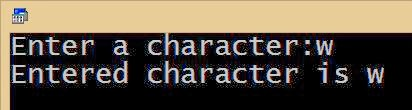# Accepting User Input using Scanf Function

In previous lesson, we learnt how to manage variables and values of them. But those values are static values, that is all values are declared by programmer during writing codes, and user has no role while running those programs.

Now let us learn how to accept a value from user by keyboard input and manage accepted values for different operation.

• We can learn with this example (Accept and show a number):
 #include   #include   void main()   {    int a;    printf(“Enter a number”);    scanf(“%d”,&a);    printf(“Entered number is %d”,a);      getch();   }

int a: : Declaring an integer variable. Variable name is ‘a’.

printf(“Enter a number”); : This line just print ‘Enter a number’, nothing more than it does. However, it indicate user to enter a number.

scanf(“%d”,&a); : This is an important line. This line accepts a number from user and stores that value to the variable ‘a’.

Here function scanf is using for reading a value from user and store to variable a. Here we have to read an integer (number) value, so we use ‘%d’ in side double quotes. Then just put a comma(,). After this, we type the variable ( here is ‘a’) to store value with an ampersand sign (&). Here is ‘&a’.

printf(“Entered number is %d”,a); : We already learnt this line of code. This code just print (display) the value on output monitor screen.

Sample output:We think you understood all above codes’ explanation

• Now let us write a program to accept and display a character.
 #include #include void main() { char c;    clrscr(); printf(“Enter a character”); scanf(“%c”,&c); printf(“Entered character is %c”,c);    getch(); }

Explanation:

char c; : Declaring a character variable ‘b’.

clrscr(); : This line clear the previous output screen.

printf(“Enter a character”); : This line just prints ‘Enter a character’. Therefore, user can understand that he can type a character now.

scanf(“%c”,&c); : This line accept a character and store to variable b. Here we have to accept a character, so we use ‘%c’. After this, just type the variable to store character with a an ampersand sign ‘&b’.

printf(“Entered character is %c”,c); : We already learnt this line of code in previous lesson. This line just print accepted character.

Sample output:• Program to accept two numbers and find their sum:
 #include #include void main() { int a,b,sum; printf(“Enter first number”); scanf(“%d”,&a); printf(“Enter second number”); scanf(“%d”,&b); sum = a + b; printf(“Sum of %d and %d is %d”,a,b,sum);    getch(); }

Explanation:

int a,b,sum; : a and b are variables used to store accepted numbers from user and variable ‘sum’ is used to store sum of a and b.

sum = a + b; : Here variable sum gets the sum of value of a and b

printf(“Sum of %d and %d is %d”,a,b,sum); : This line prints the sum of accepted numbers. First %d will replace by a and second %d will replace with b and thirst %d will replaced by the variable sum.

Sample Output:• Write a C program to accept two numbers and perform mathematical operations such that Addition (already done), subtraction, multiplication and division.

 #include #include void main() { int a,b,sum,sub,mult,div; printf(“Enter two numbers”); scanf(“%d %d”,&a,&b); sum = a + b; sub = a – b; mult = a * b; div = a / b; printf(“Sum of %d and %d is %d”,a,b,sum); printf(“Difference between %d and %d is %d”,a,b,sub); printf(“Multiplication value of %d and %d is %d”,a,b,mult); printf(“Value of %d divides %d is %d”,a,b,div); getch(); }

Explanation:
sum = a + b; : The variable sum gets the value of sum of a and b.
sub = a – b; : The variable sub gets the value of difference of a and b.
mult = a * b; : The variable mult gets the value of multiplication of a and b.
div = a / b; : The variable div gets the value a divides b.

Sample Output:• Program to find Square and cube of a number:
 #include #include void main() { int no,sqr,cub; printf(“Enter a number”); scanf(“%d”,&no); sqr = no * no ; cub =  no * no * no ; printf(“Square of %d is %d”,no,sqr); printf(“Cube of %d is %d”,no,cub); getch(); }

Explanation:

sqr = a * a; : Square of a number is the multiplying a number with same number. Therefore, we multiply value of ‘a’ (accepted number) by itself. Then store its answer to variable ‘sqr’.

cub = a * a * a; : Cube of a number is the multiplying a number with same number for two times. Therefore, we multiply value of ‘a’ (accepted number) by itself for two times. Then store its answer to variable ‘cub’.

Sample Output:• Program to find negative of given number:
 #include #include void main() { int no; printf(“Enter a number”); scanf(“%d”,&no); printf(“Negative of number %d is %d”,no,-no); getch(); }

Explanation:

Negative of a number is the multiplying that number with -1, or just putting a negative sing before given number.

If the number is 10, then its negative is  10  x -1 = – 10. That is – (number).

Sample Output: